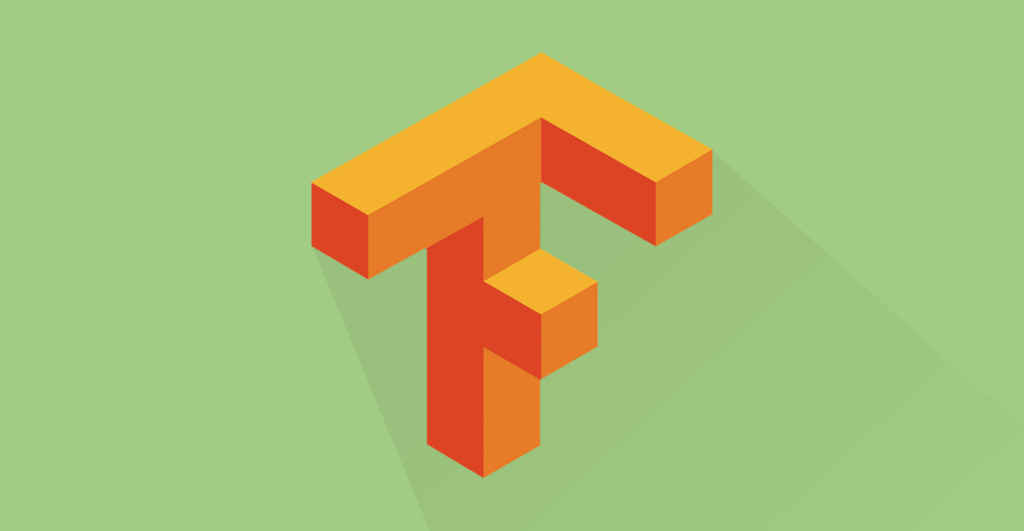## 变化

tflearn 是当时教程中我们使用的高阶深度学习框架，它基于 Tensorflow 之上，包裹了大量的细节，让用户可以非常方便地搭建自己的模型。

Tensorflow 的威力，不只在于本身构建和训练模型是不是好用。那其实只是深度学习中，非常小的一个环节。不信？你在下图里找找看。

## 环境

Colab 为你提供了全套的运行环境。你只需要依次执行代码，就可以复现本教程的运行结果了。

## 代码

``````!wget https://raw.githubusercontent.com/wshuyi/demo-customer-churn-ann/master/customer_churn.csv
``````

``````import pandas as pd
``````

``````df = pd.read_csv('customer_churn.csv')
``````

``````df.head()
``````

``````df.columns
``````

• RowNumber：行号，这个对于模型没用，忽略
• CustomerID：用户编号，这个是顺序发放的，忽略
• Surname：用户姓名，对流失没有影响，忽略
• CreditScore：信用分数，这个很重要，保留
• Geography：用户所在国家/地区，这个有影响，保留
• Gender：用户性别，可能有影响，保留
• Age：年龄，影响很大，年轻人更容易切换银行，保留
• Tenure：当了本银行多少年用户，很重要，保留
• Balance：存贷款情况，很重要，保留
• NumOfProducts：使用产品数量，很重要，保留
• HasCrCard：是否有本行信用卡，很重要，保留
• IsActiveMember：是否活跃用户，很重要，保留
• EstimatedSalary：估计收入，很重要，保留
• Exited：是否已流失，这将作为我们的标签数据

``````!pip install -q tensorflow==2.0.0-alpha0
``````

``````import numpy as np
import tensorflow as tf
from tensorflow import keras
from sklearn.model_selection import train_test_split
from tensorflow import feature_column
``````

``````tf.random.set_seed(1)
``````

``````train, test = train_test_split(df, test_size=0.2, random_state=1)
``````

``````train, valid = train_test_split(train, test_size=0.2, random_state=1)
``````

``````print(len(train))
print(len(valid))
print(len(test))
``````

``````feature_columns = []
``````

``````numeric_columns = ['CreditScore', 'Age', 'Tenure', 'Balance', 'NumOfProducts', 'EstimatedSalary']
``````

• CreditScore：信用分数
• Age：年龄
• Tenure：当了本银行多少年用户
• Balance：存贷款情况
• NumOfProducts：使用产品数量
• EstimatedSalary：估计收入

``````for header in numeric_columns:
``````

``````categorical_columns = ['Geography', 'Gender', 'HasCrCard', 'IsActiveMember']
``````
• Geography：用户所在国家/地区
• Gender：用户性别
• HasCrCard：是否有本行信用卡
• IsActiveMember：是否活跃用户

``````def get_one_hot_from_categorical(colname):
categorical = feature_column.categorical_column_with_vocabulary_list(colname, train[colname].unique().tolist())
return feature_column.indicator_column(categorical)
``````

``````geography = get_one_hot_from_categorical('Geography'); geography
``````

``````for col in categorical_columns:
feature_columns.append(get_one_hot_from_categorical(col))
``````

``````feature_columns
``````

6个数值类型，4个类别类型，都没问题了。

``````from tensorflow.keras import layers
``````

``````feature_layer = layers.DenseFeatures(feature_columns); feature_layer
``````

`relu` 函数大概长这个样子：

``````model = keras.Sequential([
feature_layer,
layers.Dense(200, activation='relu'),
layers.Dense(100, activation='relu'),
layers.Dense(1, activation='sigmoid')
])
``````

`sigmoid` 函数的长相是这样的：

``````model.compile(optimizer='adam',
loss='binary_crossentropy',
metrics=['accuracy'])
``````

``````def df_to_tfdata(df, shuffle=True, bs=32):
df = df.copy()
labels = df.pop('Exited')
ds = tf.data.Dataset.from_tensor_slices((dict(df), labels))
if shuffle:
ds = ds.shuffle(buffer_size=len(df), seed=1)
ds = ds.batch(bs)
return ds
``````

``````train_ds = df_to_tfdata(train)
valid_ds = df_to_tfdata(valid, shuffle=False)
test_ds = df_to_tfdata(test, shuffle=False)
``````

``````model.fit(train_ds,
validation_data=valid_ds,
epochs=5)
``````

``````model.summary()
``````

``````model.evaluate(test_ds)
``````

……

• 训练集损失
• 训练集准确率
• 验证集损失
• 验证集准确率

## 小结

1. Tensorflow 2.0 的安装与使用；
2. 表格式数据的神经网络分类模型构建；
3. 特征工程的基本流程；
4. 数据集合的随机分割与利用种子数值保持一致；
5. 数值型数据列与类别型数据列的分别处理方式；
6. Keras 高阶 API 的模型搭建与训练；
7. 数据框转化为 Tensorflow 数据流；
8. 模型效果的验证；
9. 缺失的一环，也即本文疑点产生的原因，以及正确处理方法。